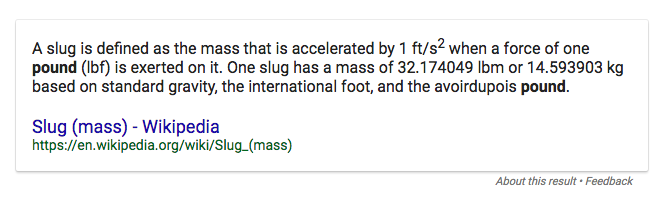## Sunday, March 26, 2017

### Units, Variables, and Formulas

When physics formulas are considered, there are many letters to think about. The formulas, themselves, are a string of variables represented by letters. Each letter represents some quantity, and the formula shows how the quantities are related to each other.

In some formulas, the displacement is considered. Displacement (and also distance) is represented by the letter d. However, many times, it is necessary to look at multiple displacements in the same situation, subscripts are used for initial and final displacements: di and df. Often, for convenience, the subscript is displayed in the same size as the variable resulting in di and df.

In other formulas, the rate that displacement changes (velocity) is considered. Velocity is represented by the letter v. However, many times, it is necessary to look at multiple velocities in the same situation, subscripts are used for initial and final displacements: vi and vf. As with displacement, for convenience, the subscript is displayed in the same size as the variable resulting in vi and vf

When formulas consider the rate at which velocity changes (acceleration), the letter a is used.

When working with acceleration, it is sometimes necessary to look at an average velocity, which is represented as v(ave) or just v(ave). Graphically, it can be written as a v with a line on top.

Elapsed time is represented by the letter t. (It is sometimes necessary to find elapsed time by taking the difference between two clock readings, which are called—and which are confusing—initial time and final time, ti and tf).

Formulas using force will represent for with an F (capital). Working with forces often requires considering multiple forces all at once. Thus, net force becomes Fnet and the component forces get subscripted with numbers such as F1 or F2. As in other cases, for typing convenience, the subscript is sometimes rendered in the same size font as the variable.

One more variable that relates to force and acceleration is mass, and it is represented by an m. If necessary to designate different masses in the same situation, subscripts are used to designate which is which.

The following formulas give examples of how the variables might appear in equations.

df = di + vit + 1/2at2

vf = vi + at

F = ma

v(ave) = (vi + vf)/2

One challenge to solving physics problems is working the given information into the equations. Paying attention to the units is the key to doing this successfully. The following guide will help identify where quantities with specific units should go.

Distances — df, di, d — units will be something that measures distance, such as meters (m), miles (mi), feet (ft), inches (in), centimeters (cm), etc.
Examples:
3 ft
2 m
34 cm

Time — t — units will be something that measures time, such as hours (hr), minutes (min), or seconds (s).
Examples:
3 s
4 min
65 hr

Velocity — vf, vi, v — units will be some distance unit divided by some time unit, as shown in the examples.
8 m/s
398 cm/s
4 mph
67 MPH
11 mi/s
39 ft/min

Acceleration — a — will be some velocity unit divided by some time unit, as shown in the examples.
Examples:
3 (m/s)/s
4 MPH/s
38 (m/s)/s

Force — F, Fnet, F1, F2 — will be in newtons, a derived unit of (kg•(m/s))/s. Force should also have a direction given.
Examples:
3 N Up
2 N LeftMass — m — units will be a mass unit, such as grams (g or gm), kilograms (kg) or slugs (never mind!)
Examples:
3 gm
5 kg